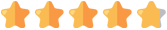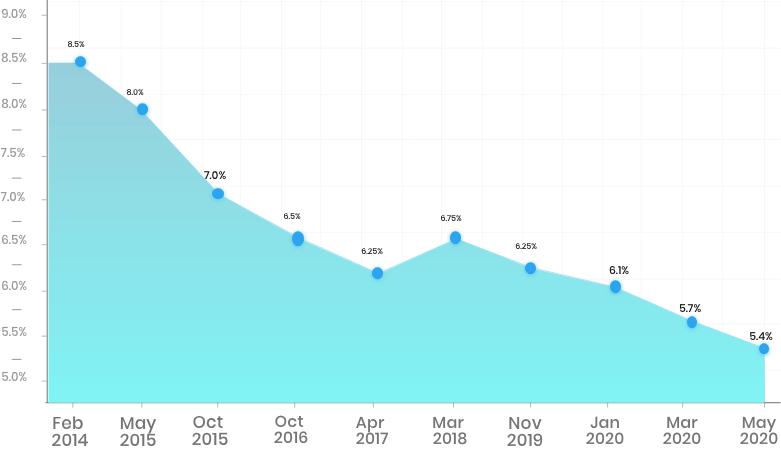# PNB Fixed Deposit (FD) Interest Calculator

PNB FD rates calculator is a free online tool that helps people estimate the maturity amount from the fixed deposit investment in PNB savings accounts. Within seconds, you can find out the amount gained using the PNB rates FD calculator. FD calculator is a tool provided to get an idea about the maturity amount that the investor ought to expect towards the finish of a decided tenure for a predefined deposit at the appropriate rate of interest.

Best Investment Options
• Save upto ₹46,800 in tax under Sec 80C

• Inbuilt Life Cover

• Tax Free Returns Unlike FD

*All savings are provided by the insurer as per the IRDAI approved insurance plan. Standard T&C Apply

Get Guaranteed returns along with life cover
invest in 100% Guaranteed Return Plans Tax benefits under sec 80C & No Tax on returns*
+91
View Plans
Plans available only for people of Indian origin By clicking on "View Plans", you agree to our Privacy Policy and Terms of Use #For a 55 year on investment of 20Lacs #Discount offered by insurance company Tax benefit is subject to changes in tax laws
We are rated58.9 million
Registered Consumers
51
Insurance
Partners
26.4 million
Policies
Sold
PNB Bank FD Calculator

Total Investment

₹500 ₹10L
Enter Total Investment

Rate of Interest (Yearly)

1% 10%
Rate of Interest (Yearly)

Time Period

1 Year 10 Years
Enter Time Period
Total Investment
Interest Earned
Maturity Amount

## How can PNB Bank FD Calculator Help?

The PNB Bank FD calculator is accessible online, and anyone can utilize it free of cost. This calculating tool helps in assessing the maturity worth of an FD or a fixed deposit investment. One needs to enter a couple of essential details like the rate of interest and the investment sum.

To check how much interest your fixed deposit will acquire toward the finish of the selected tenure, you simply need to give the essential details of your FD account, for example, investment sum, term of deposit and rate of interest.

It gives the details of speculation upon development after the interest is accumulated on days or a month to month, quarterly, half-yearly and yearly premise.

This calculating tool will assist you with ascertaining the interest that your chief sum will procure when kept in a PNB fixed deposit account.

Like any remaining banks, the interest rates on PNB FD can likewise be determined by consistently utilizing a PNB FD calculator.

PNB offers various FD choices to its clients with attractive interest rates.

## Working of a PNB Bank FD Calculator

If a record holder picks the occasional interest payout alternative option, PNB moves the interest procured at each interval, for instance, quarterly, to their bank account. The interest would remain the same as before for the duration of the fixed deposit bank account tenure. On development, PNB would move the investment amount up to your bank account, except if the deposit is restored.

The Punjab National Bank FD calculator makes it simple to estimate the interest you will procure on your PNB FD account.

## Factors Affecting the Maturity Amount Interest Rate

It is the rate at which the profits are accumulated on the contributed sum. Note that the interest rates shift with the term of deposit of your FD.

• Timespan: very much like the investment sum and the rate of interest, the term of your FD is likewise one of the important components. The bank presets the rate of interest of your sum depending on the FD tenure.
• Investment sum: It is the primary fundamental consideration. The interest is procured on the principal sum, which is the sum kept by you at the beginning of opening the fixed deposit bank account.
• Accumulating or compounding frequency: compounding or accumulative frequencies implies the frequency at which the interest is determined on a fixed deposit sum. It very well may be accumulated quarterly, month to month, yearly and half-yearly. This term of the deposit is instrumental in deciding the maturity amount on the fruition of the period of the term deposits.*All savings are provided by the insurer as per the IRDAI approved insurance plan. Standard T&C Apply

## Simple Interest vs. Compound Interest

Simple interest depends on the principal sum of the deposit. Conversely, compound interest depends on the principal sum and the interest gathered in each period.

Compound interest

This is the interest procured on the sum as well as its interests. It is determined by the product of the sum with the rate of interest raised to the power of the number of periods in years for which the interest has to be computed.

If you are considering how to figure the compound interest on PNB fixed deposit sum, you ought to likely note down the equation to calculate the interest acquired on PNB FD, which is

A = P (1 + r/n) ^ (n*t)

Here,

A is Amount at Maturity

P is the Amount of Investment

r is the Rate of Interest

n is the number of times of compounding the interest

t is Investment term

For example, if a sum of Rs 10,000 is at 10% compound interest rate invested for 3 years. The interest is to be compounding quarterly.

So,

Amount= 10,000 {1 + (0.1/4)} ^ (4 * 3)

A = 10,000 (1 + 0.025) ^ (12)

A = 10,000 (1.025) ^ (12) = Rs. 13,449 (approximately)

So, now, the Compound Interest earned will be = Maturity Amount – Principal Amount

i.e., 13,449 – 10,000 = Rs. 3,449

Simple interest

Simple interest is the interest procured on investment at a pre-chosen interest rate for a particular time frame. It is determined by multiplying the deposit sum, the rate of interest per annum and the time for which the cash is lent in years.

SI = (P x R x T)/ 100

Where,

SI is Simple Interest

P is Principal (amount invested)

R is the Rate of Interest (in %)

T is Tenure, the period for which deposit is kept in FD account.

For the same example,

If a sum of Rs 10,000 is invested for 3 years at 10% simple interest rate, then :

Interest= (10000* 3* 10)/100 = 3000.

Amount= 10,000+3000 = 13,000.

FD interest have fallen consistently over the last 5 years.
Invest in Plans that offer Guaranteed Returns for over 25 Years
Know More## Advantages of Using PNB Bank Fixed Deposit Calculator

An FD calculator is a tool that figures the amount one will get on investing sum into a fixed deposit (FD) at the time of development. This tool utilizes inputs like the sum, period of the FD and the predominantly fixed deposit rate for the decided tenure.

Anything that saves your time and efforts on complex estimations is constantly involved for certain advantages, and the Fixed Deposit calculator is not a special case. Below given are the advantages of the PNB FD rate calculator:

• The calculating tool is free of cost; hence, clients can utilize it on various occasions and look at returns for mismatched combinations of FD rates, tenure, and sum.
• You can calculate the specific development worth of your FD simply by giving essential details, for example, the investment sum, rate of interest, period of the venture and kind of interest accumulating recurrence.
• Easy to utilize from its easy to use interface
• No extent of mistakes since it's a programmed calculator
• Calculator precisely appraises the profits that one may procure from PNB FDs.## How to Use a PNB Fixed Deposit Calculator?

The PNB FD calculator works utilizing the fundamental data expected to calculate the ideal result. The procedure is straightforward and easy to use at the bank portal. A comparable standard is utilized at the PNB Bank FD calculator to help the financial model make a reasonable deposit. The steps included are distinct and basic for any nonprofessional to utilize and to decide on investments addressing individual requirements. The following steps are:

• Visit the PNB portal and invoke the FD calculator.
• Select thePNB Bank FD Calculator.
• Insert the principal amount or sum.
• Select the investment tenure.
• Pick the kind of deposit options, whether reinvestment, quarterly, or regularly scheduled payout.
• Click on the calculate button to get the outcome displayed.

There is no limit on the number of times the calculating tool is utilized to assess the FD returns on investments.*All savings are provided by the insurer as per the IRDAI approved insurance plan. Standard T&C Apply

## FAQs

• ### Q1. Is it free to use the FD calculator at PNB?

A1. Yes. The FD calculator available at PNB is free to be used anytime.

• ### Q2. Does a PNB FD calculator request different things aside from the fundamental details?

A2. No, the Punjab Public Bank will ask you just the essential details relating to your account, for example, the deposit sum, rate of interest, period, and accumulating frequency.

• ### Q3. How does the PNB FD Calculator produce the ideal output?

A3. The calculating tool factors in the tenure. For under a half year, simple interest is applied, while for a longer period, quarterly compounding guidelines are applied.

• ### Q4. Is the interest acquired on PNB fixed deposits taxable?

A4. Indeed, the interest procured on FDs will be taxed just if the absolute interest earned in a given monetary year surpasses Rs. Rs.10, 000.

• ### Q5. Can I withdraw prematurely from my FD account in PNB?

A5. PNB allows its customers to withdraw prematurely on the FDs. Nonetheless, one gets charged a penalty for withdrawing early. PNB deducts a 1% penalty before paying the financial investor the due interest on maturity.

Disclaimer: Policybazaar does not endorse, rate or recommend any particular insurer or insurance product offered by an insurer.Disclaimer: Policybazaar does not endorse, rate or recommend any particular insurer or insurance product offered by an insurer.
CalculatorsClose